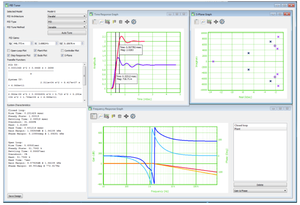# SIAD System ID, Analysis and Design Software

## Featured Product from Venable InstrumentsSIAD is a complete software package designed to easily estimate the transfer function from any measured frequency response data, translate it into the time domain, analyze the transient response, and tune the PID compensation model.

SIAD Software can estimate a mathematical model (Transfer Function) for plant (power stage), use this model to tune the PID compensation (feedback) and plot the open and closed loop gain of the frequency response in one integrated application system.

SIAD provides a system identification tool to quickly estimate and graphically validate the transfer function from the imported data. This tool allows the users to enter the number of poles and zeros in order to estimate the transfer function.

The software can calculate the characteristics of the system model and provides graphs such as bode, s-plane and step response graphs to analyze the system model.

SIAD utilizes Ziegler-Nichols (mathematical calculation) as well as the Venable PID tuning tools (curve fitting algorithm) to automatically adjust the PID coefficients of controller.

Frequency Response Analyzer (FRA) data from any FRA can be imported as a VEN, TXT, CSV, or MATLAB ® file formats, allowing the user to move from data to analysis seamlessly.

Key Features

• Import frequency response measurement data from VEN, TXT, CSV, and MATLAB files.
• Estimating the transfer function (mathematical model) from frequency response data.
• Provides different plots for model analysis.
• Calculating the characteristics of the system model.
• Providing PID tuning tools to design compensation and model the results.
• Easy graphical user interface to quickly understand and use the software